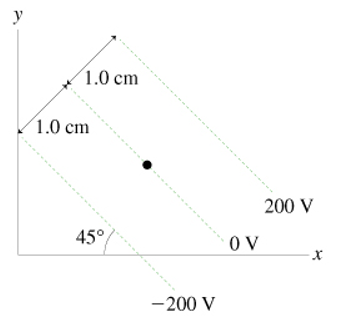# Problem: Part A. What is the magnitude of the electric field at the dot in the figure? (Figure 1) Express your answer using two significant figures. Part B. What is the direction of the electric field at the dot in the figure?A. the negative x-axisB. the positive x-axisC. 45° below -x axisD. 45° below +x-axis

###### FREE Expert Solution

The electric field (E) as a derivative of the potential difference (ΔV) is expressed as:

$\overline{){\mathbf{E}}{\mathbf{=}}\frac{\mathbf{∆}\mathbf{V}}{\mathbf{d}}}$, where d is the separation between two points.

100% (66 ratings)###### Problem DetailsPart A. What is the magnitude of the electric field at the dot in the figure? (Figure 1) Express your answer using two significant figures.

Part B. What is the direction of the electric field at the dot in the figure?

A. the negative x-axis

B. the positive x-axis

C. 45° below -x axis

D. 45° below +x-axis